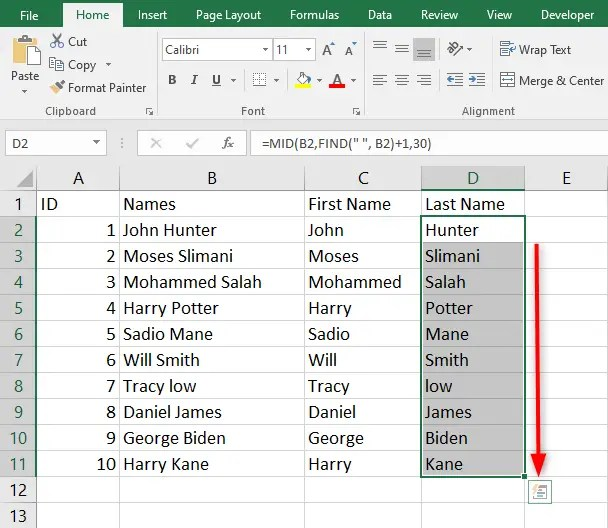# How to FIND MID in Excel

Excel’s MID Function is categorized under Excel TEXT Functions. The MID Formula returns a specified value of characters from the middle of a given text string. The function requires three arguments which include: the n numbers of characters to return, text to extract from the original text string, and a start point. Generally, you can manipulate text strings using the MID Function combined with other functions such as SUM, DATE, VALUE, COUNT, DAY, and many others.

In this article, we discuss how to find MID in Excel. Let’s get started.

## The MID Function formula

`=MID (text, start_num, num_chars)`

The MID Function arguments explained:

1. Text – this is a required argument that specifies the original text string.
2. Start_num – this is a required argument that is a number that shows the position of the first character that you wish to extract.
3. Num_chars – it is a required argument that specifies the number of characters. It starts with start_num, which is to be returned from the start of a given text string. In short, Num_chars represents the number of characters to be extracted.

### Steps to take to find MID in Excel

MID is a worksheet function that can be used partly as a formula in a cell of a worksheet.

For example:

Let’s use the MID Function that returns 3 characters starting at the 5th from the text string below:

The egg in the cup

Your formula will look like this:

`=MID ("The egg in the cup", 5, 3)`

The formula will return “egg” as the answer as it meets the formula’s criteria.But, when using the same example above and looking for 3 characters starting at character 16, the formula will return “cup” as the result. Here is what your formula will look like:

`=MID ("The egg in the cup", 16, 3)`

## How to extract the first and last name using the FIND MID function

You can extract the first name from a string using the the FIND MID function.  . For example, let’s say the full name is in cell B4, where the first and last names are separated with a space character. To pull the first name using the MID formula, here is what you will do:

`=MID(B2,1,FIND(" ",B2))`To get the last name, your formula will be like this:

`=MID(B2,FIND(" ", B2)+1,30)`Drag the fill handles to fill the remaining cells automaticallyIn both instances, the FIND function determines the starting position.

Mid function template

## Important things to know about the MID Function

MID formula returns a text string even if the extracted string contains digits. In case you need to use value results, convert the output into a number using the MID function combined with the VALUE function.

In cases where start_num is less than 1, Excel’s MID function returns the #VALUE! Error.

In cases where num_chars is a negative number (less than zero), Excel’s MID function returns #VALUE! Error. But if num_chars is equal to zero, you get an empty string as the output results.